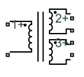# Three-Winding Transformer

Compute sum of RF signals

• Library:
• RF Blockset / Circuit Envelope / Elements

•## Description

The Three-Winding Transformer block models three coupled inductors within the circuit envelope simulation environment.

The block implements the relations

`$\begin{array}{c}{v}_{1}\left(t\right)={L}_{1}\frac{d}{dt}\left[{i}_{1}\left(t\right)\right]+{M}_{12}\frac{d}{dt}\left[{i}_{2}\left(t\right)\right]+{M}_{13}\frac{d}{dt}\left[{i}_{3}\left(t\right)\right]\\ {v}_{2}\left(t\right)={M}_{12}\frac{d}{dt}\left[{i}_{1}\left(t\right)\right]+{L}_{2}\frac{d}{dt}\left[{i}_{2}\left(t\right)\right]+{M}_{23}\frac{d}{dt}\left[{i}_{3}\left(t\right)\right]\\ {v}_{3}\left(t\right)={M}_{13}\frac{d}{dt}\left[{i}_{1}\left(t\right)\right]+{M}_{23}\frac{d}{dt}\left[{i}_{2}\left(t\right)\right]+{L}_{3}\frac{d}{dt}\left[{i}_{3}\left(t\right)\right]\\ {M}_{pq}={K}_{pq}\sqrt{{L}_{p}{L}_{q}}\end{array}$`

where:

• L1, L2, and L3 represent inductances.

• Mpq represents the mutual inductance between the pth and qth inductors, with coefficient of coupling Kpq.

• v1(t), v2(t), and v3(t) represent the voltage across the terminals of the inductors at time t.

• i1(t), i2(t), and i3(t) represent the current through the inductors at time t. The block uses standard dot notation to indicate the direction of positive current flow relative to a positive voltage.

RF Blockset™ current and voltage signals consist of in-phase (Ik) and quadrature (Qk) components at each frequency fk specified in the Configuration block:

`$\begin{array}{c}i\left(t\right)=\sum _{\left\{{f}_{k}\right\}}\left({i}_{{I}_{k}}\left(t\right)+j\cdot {i}_{{Q}_{k}}\left(t\right)\right){e}^{j\left(2\pi {f}_{k}\right)t}\\ v\left(t\right)=\sum _{\left\{{f}_{k}\right\}}\left({v}_{{I}_{k}}\left(t\right)+j\cdot {v}_{{Q}_{k}}\left(t\right)\right){e}^{j\left(2\pi {f}_{k}\right)t}\end{array}$`

## Parameters

expand all

Inductance value of the first inductor, specified as a nonnegative scalar integer in henries. Specify the units of the inductance from the corresponding drop-down menu.

Data Types: `double`

Inductance value of the second inductor, specified as a nonnegative scalar integer in henries. Specify the units of the inductance from the corresponding drop-down menu.

Data Types: `double`

Inductance value of the third inductor, specified as a nonnegative scalar integer in henries. Specify the units of the inductance from the corresponding drop-down menu.

Data Types: `double`

Inductance value of the third inductor, specified as a nonnegative scalar integer in henries. Specify the units of the inductance from the corresponding drop-down menu.

Data Types: `double`

Coupling coefficient for the mutual inductance of the first and second inductors, specified as a nonnegative scalar integer between `0` and `1`

Data Types: `double`

Coupling coefficient for the mutual inductance of the first and third inductors, specified as a nonnegative scalar integer between `0` and `1`

Data Types: `double`

Coupling coefficient for the mutual inductance of the second and third inductors, specified as a nonnegative scalar integer between `0` and `1`

Data Types: `double`

Note

The minimum nonzero inductance value that the RF Blockset environment recognizes is `1e-18` `H`. During simulation, the block uses a value of `1e-18` `H` for any inductance and mutual inductance values specified between `0` and `1e-18` `H`.

## Version History

Introduced before R2006a